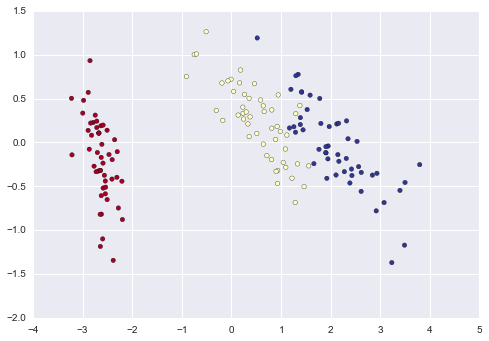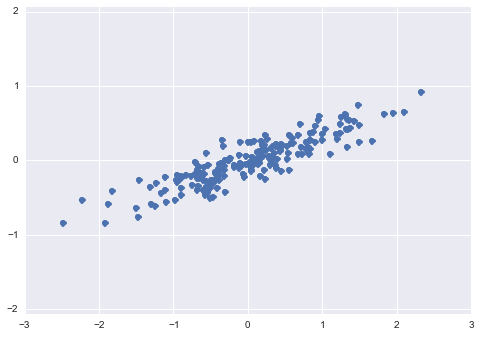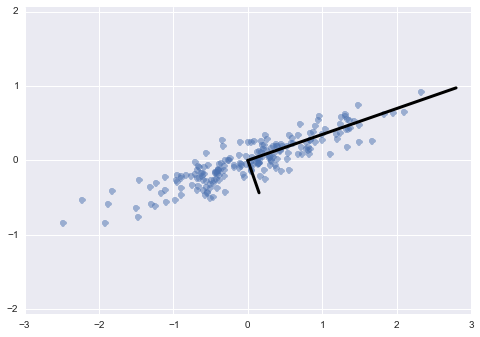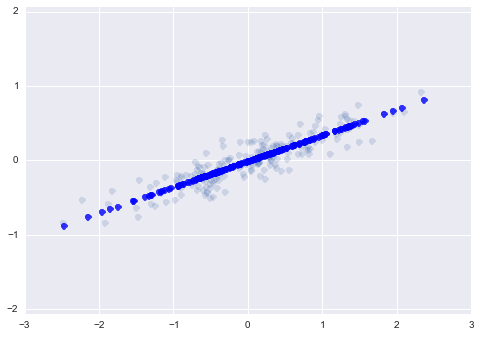# scikit-learn-pca¶

Credits: Forked from PyCon 2015 Scikit-learn Tutorial by Jake VanderPlas

## Dimensionality Reduction: PCA¶

In :
%matplotlib inline
import numpy as np
import matplotlib.pyplot as plt
import seaborn;
from sklearn import neighbors, datasets

import pylab as pl

seaborn.set()

X, y = iris.data, iris.target
from sklearn.decomposition import PCA
pca = PCA(n_components=2)
pca.fit(X)
X_reduced = pca.transform(X)
print("Reduced dataset shape:", X_reduced.shape)

import pylab as pl
pl.scatter(X_reduced[:, 0], X_reduced[:, 1], c=y,
cmap='RdYlBu')

print("Meaning of the 2 components:")
for component in pca.components_:
print(" + ".join("%.3f x %s" % (value, name)
for value, name in zip(component,
iris.feature_names)))

('Reduced dataset shape:', (150, 2))
Meaning of the 2 components:
0.362 x sepal length (cm) + -0.082 x sepal width (cm) + 0.857 x petal length (cm) + 0.359 x petal width (cm)
-0.657 x sepal length (cm) + -0.730 x sepal width (cm) + 0.176 x petal length (cm) + 0.075 x petal width (cm)# Dimensionality Reduction: Principal Component Analysis in-depth¶

Here we'll explore Principal Component Analysis, which is an extremely useful linear dimensionality reduction technique. Principal Component Analysis is a very powerful unsupervised method for dimensionality reduction in data. Look for directions in the data with the most variance.

Useful to explore data, visualize data and relationships.

It's easiest to visualize by looking at a two-dimensional dataset:

In :
np.random.seed(1)
X = np.dot(np.random.random(size=(2, 2)), np.random.normal(size=(2, 200))).T
plt.plot(X[:, 0], X[:, 1], 'o')
plt.axis('equal');We can see that there is a definite trend in the data. What PCA seeks to do is to find the Principal Axes in the data, and explain how important those axes are in describing the data distribution:

In :
from sklearn.decomposition import PCA
pca = PCA(n_components=2)
pca.fit(X)
print(pca.explained_variance_)
print(pca.components_)

[ 0.75871884  0.01838551]
[[ 0.94446029  0.32862557]
[ 0.32862557 -0.94446029]]

In :
plt.plot(X[:, 0], X[:, 1], 'o', alpha=0.5)
for length, vector in zip(pca.explained_variance_ratio_, pca.components_):
v = vector * 3 * np.sqrt(length)
plt.plot([0, v], [0, v], '-k', lw=3)
plt.axis('equal');Notice that one vector is longer than the other. In a sense, this tells us that that direction in the data is somehow more "important" than the other direction. The explained variance quantifies this measure of "importance" in direction.

Another way to think of it is that the second principal component could be completely ignored without much loss of information! Let's see what our data look like if we only keep 95% of the variance:

In :
clf = PCA(0.95) # keep 95% of variance
X_trans = clf.fit_transform(X)
print(X.shape)
print(X_trans.shape)

(200, 2)
(200, 1)


Isomap: manifold learning, good when PCA doesn't work like in a loop. Large number of datasets, can use randomized PCA.

By specifying that we want to throw away 5% of the variance, the data is now compressed by a factor of 50%! Let's see what the data look like after this compression:

In :
X_new = clf.inverse_transform(X_trans)
plt.plot(X[:, 0], X[:, 1], 'o', alpha=0.2)
plt.plot(X_new[:, 0], X_new[:, 1], 'ob', alpha=0.8)
plt.axis('equal');The light points are the original data, while the dark points are the projected version. We see that after truncating 5% of the variance of this dataset and then reprojecting it, the "most important" features of the data are maintained, and we've compressed the data by 50%!

This is the sense in which "dimensionality reduction" works: if you can approximate a data set in a lower dimension, you can often have an easier time visualizing it or fitting complicated models to the data.